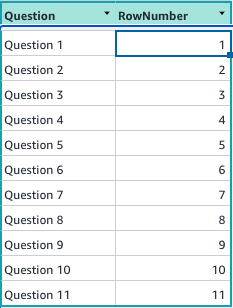# Select a random cell in a table column

Hi! New to Honeycode. Love it!

I am working on some flashcards. I have a table with questions and would like to select a different cell in the column each time I hit a button on the app.

I have tried a couple of techniques - I was looking for something like RANDBETWEEN which i don't think exists to do a random selection in a column.

So far, i added a column of RAND() numbers (column called qRAND, column B) to the questionTable and have a button that overwrites that column each time it is pushed. If I put a table on my app, i can see the qRAND fields change. My thinking was to create a filter that sorted by that RAND() column and then select cell A2 in the filter in the question column to put into a cell filed on the form.

I have tried an INDEX of FILTERS but can't seem to get the syntax right.

=INDEX(FILTER(questionTable,"ORDER BY questionTable[qRAND] ASC"),2,1)

I don't know how to get the INDEX range declared.

I am of course open to any other ideas! Still learning and went through the commnity descriptions for FILTER, PICKLISTS, INDEX, etc. Also a very good chat here: Order/sort Drop Down but i seem to be stuck.

Appreciate any guidance.

Hi @h3atmisr,

If I understand you correctly, you would like to display a random question to the user in an app.

One way to accomplish this is to use FINDROW() with a WHERE clause that selects a random row based on say a RowNumber column in a table.

=FINDOW(Questions, "Questions[RowNumber]=%", RandomQuestionNumber)

We will need to determine how to calculate the RandomQuestionNumber above.

Let's say you have a table such as the following:You can then add a Data Cell on top of the screen with the following formula:

``````=ROUND(RAND()*(MAX(Questions[RowNumber])-1)+1,0)
``````

The above formula will evaluate to a number that is between 1 and the maximum value in the RowNumber column (11 in this example). Let's say the name of this cell is RandomQuestionNumber - and its value can be referred to on the screen using \$[RandomQuestionNumber].

Once you have this random question number, you can then use FindRow to find the row whose RowNumber is this random number. You can do this by adding yet another Data Cell whose value evaluates to a row in the Question table:

=FINDROW(Questions,"Questions[RowNumber]=%",\$[RandomQuestionNumber])

Let's say we call this Data Cell CurrentQuestion - and we can refer to this as \$[CurrentQuestion]

Once this is done, you can set the source of the Block to \$[CurrentQuestion] and display any columns from this row in the Questions table.

Hope this helps.

2 Likes

Hi, You can also use RAND() instead of RANDBETWEEN to achieve similar results by multiplying the random number generated with the max value which in this case is the number of rows in the table:

=INDEXROW(questionTable,INT(RAND()*ROWS(questionTable)))

Where ROWS() returns the numbers of rows from a table or filter and INT() rounds up the number.

Love this thinking - this will be useful as i need to nest some stuff. Will look at it more! thanks!

1 Like

This worked out of the box for the button - didn't know about INDEXROW - thanks for the idea...and the syntax and explanation! Perfect!

1 Like

This topic was automatically closed 3 days after the last reply. New replies are no longer allowed.